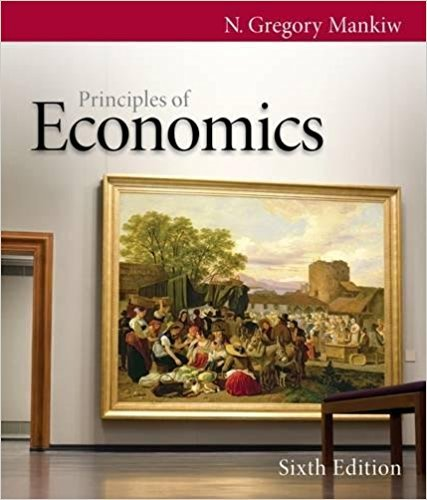×
Get Full Access to Principles Of Economics - 6 Edition - Chapter 26 - Problem Problems and applications 26.5
Get Full Access to Principles Of Economics - 6 Edition - Chapter 26 - Problem Problems and applications 26.5

×

Textbooks / Business / Principles of Economics 6 / Chapter 26 / Problem Problems and Applications 26.5

# Economists in Funlandia, a closed economy, have collected the following informationISBN: 9780538453059 472

## Solution for problem Problems and Applications 26.5 Chapter 26

Principles of Economics | 6th Edition

• Textbook Solutions
• 2901 Step-by-step solutions solved by professors and subject experts
• Get 24/7 help from StudySoup virtual teaching assistantsPrinciples of Economics | 6th Edition

4 5 1 245 Reviews
26
5
Problem Problems and Applications 26.5

Economists in Funlandia, a closed economy, have collected the following information about the economy for a particular year: Y = 10,000 C = 6,000 T = 1,500 G = 1,700 The economists also estimate that the investment function is: I = 3,300 100 r, where r is the countrys real interest rate, expressed as a percentage. Calculate private saving, public saving, national saving, investment, and the equilibrium real interest rate.

Step-by-Step Solution:
Step 1 of 3

Wednesday, October 5, 2016 Wireless Communication in Business MIS 111 - http:// • Deﬁning for the browser how to transfer information • Protocol - Challenges of Wireless Communication • Conﬁdentiality • Integrity • Accessibility - Decreasing Signal Strength • radio signal attenuates as it propagates through matter (path loss) - Interference from Other Sources • un regulated wireless network frequencies shared by other devices and general noise source - Multi-path propagation • radio signal reﬂects off objects ground, arriving at destination at slightly different times - Wireless Transmission Media • Wireless transmission over unguided media (air) • Decreasing signal strength (distance) • Interference (noise) • Multi-path (e

Step 2 of 3

Step 3 of 3

##### ISBN: 9780538453059

The answer to “Economists in Funlandia, a closed economy, have collected the following information about the economy for a particular year: Y = 10,000 C = 6,000 T = 1,500 G = 1,700 The economists also estimate that the investment function is: I = 3,300 100 r, where r is the countrys real interest rate, expressed as a percentage. Calculate private saving, public saving, national saving, investment, and the equilibrium real interest rate.” is broken down into a number of easy to follow steps, and 70 words. The full step-by-step solution to problem: Problems and Applications 26.5 from chapter: 26 was answered by , our top Business solution expert on 03/16/18, 04:26PM. This full solution covers the following key subjects: . This expansive textbook survival guide covers 36 chapters, and 670 solutions. Since the solution to Problems and Applications 26.5 from 26 chapter was answered, more than 436 students have viewed the full step-by-step answer. Principles of Economics was written by and is associated to the ISBN: 9780538453059. This textbook survival guide was created for the textbook: Principles of Economics, edition: 6.

Unlock Textbook Solution